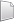# HS-1036: Set Up an Excel Model with a Visual Basic Script

Learn how to couple HyperStudy with a spreadsheet containing Visual Basic scripts and identify input variables and output responses.

Before you begin, copy the model files used in this tutorial from <hst.zip>/HS-1036/ to your working directory.

When you create an Excel spreadsheet model, it is important that the spreadsheet is formatted correctly. A variable's value and label can be formatted in two consecutive rows or two consecutive columns. Variable labels should only contain English characters, or a combination of English characters and numbers. If a label is not created for a variable, HyperStudy will assign one by default.

1. In Excel, open the iBeam_withMacros.xls file.
This spreadsheet performs calculation for a top loaded cantivlevered beam with an “I” cross section. The input variables and output responses are labeled for clarity.
2. In the cell to the right of Web Thick, change the value.
3. Click Run macro named PerformCalcs to update the calculated output responses.
The formulas and operations to calculate the output response values are contained in a Visual Basic Script macro called “Perform Calculations”. When a change is made to one of the input variables, it is not reflected in the output responses until you run the script.
Note: To run the Visual Basic script macro, you must enable macros in Excel.

## Perform the Study Setup

1. Start HyperStudy.
2. Start a new study in the following ways:
• From the menu bar, click File > New.
• On the ribbon, click.
3. In the Add Study dialog, enter a study name, select a location for the study, and click OK.
4. Go to the Define Models step.
1. From the Directory, drag-and-drop the iBeam_withMacro.xls file into the work area.
The Solver input file field displays hst_input.hstp, this is the name of the solver input file Summary panel writes during an evaluation.
2. In the Solver input arguments column, enter PerformCalcs.
This is the name of the Visual Basic script.
6. Click Import Variables.
1. In the Excel dialog, click Yes to begin selecting input variables.
2. In the spreadsheet, select the cells that contain the input variable's labels and values.
3. In the Excel dialog, click OK.
4. Click Cancel to stop selecting input variables.
1. In the Excel dialog, click Yes to begin selecting output responses.
2. In the spreadsheet, select the cells that contain the output response's labels and values.
3. In the Excel dialog, click OK.
4. Click Cancel to stop selecting output responses.
Four input variables and four output responses are imported from the iBeam_withMacro.xls spreadsheet.
9. Go to the Define Input Variables step.
10. Review the input variable's lower and upper bound ranges.

## Perform Nominal Run

1. Go to the Test Models step.
2. Click Run Definition.
An approaches/setup_1-def/ directory is created inside the study Directory. The approaches/setup_1-def/run__00001/m_1 directory contains the input file, which is the result of the nominal run.

## Review Output Responses

1. Go to the Define Output Responses step.
2. Review the output responses imported into the study.